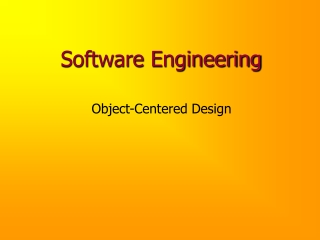DownloadDownload PresentationSoftware Engineering

# Software Engineering

Télécharger la présentation## Software Engineering

- - - - - - - - - - - - - - - - - - - - - - - - - - - E N D - - - - - - - - - - - - - - - - - - - - - - - - - - -
##### Presentation Transcript

1. Software Engineering Object-Centered Design

2. Problem Solving Does anyone scuba dive? Let’s solve this scuba-diving problem: Write a program that, given a depth under water, computes the pressure at that depth.

3. Behavior A. Describe the desired behavior of the program: Our program should display a prompt for the depth on the screen, read the depth from the keyboard, compute the corresponding pressure, and display the pressure, along with a descriptive label on the screen.

4. Objects B. Identify the nouns in the behavioral description (other than program and user): Our program should display a prompt for the depth on the screen, read the depth from the keyboard, compute the corresponding pressure, and display the pressure, along with a descriptive label on the screen. These make up the objects in our program.

5. Operations C. Identify the verbs in the behavioral description: Our program should display a prompt for the depth on the screen, read the depth from the keyboard, compute the corresponding pressure, and display the pressure, along with a descriptive label on the screen. These make up the operations in our program.

6. Algorithm D. Organize the objects and operations into a sequence of steps that solves the problem, called an algorithm. 0. Display a prompt for depth on the screen. 1. Read depth from the keyboard. 2. Compute pressure from depth. 3. Display pressure, plus an informative label on the screen.

7. Coding Once we have designed an algorithm, the next step is to translate that algorithm into a high level language like C++. This involves figuring out how to • represent our objects, and • perform our operations, in C++ (with the aid of a book, if necessary...)

8. Representing Objects A. Determine a type and name for each object:

9. Performing Operations B. Identify the C++ operator to perform a given operation, if there is one... To compute pressure, we need to find the pressure-depth formula in a reference book...

10. Depth-to-Pressure In a reference book, we find that • pressure is 0 atmospheres at depth 0 • pressure increases 1 atmosphere every 33 feet. • 1 atmosphere of pressure = 14.7 lbs./sq.in. Computing the pressure thus adds new objects and operations to our problem...

11. Objects (Revised)

12. Operations (Revised)

13. Documentation Always begin a file with an openingcomment: /* pressure.cpp is a scuba-diving utility. * Author: J. Adams * Date: 1/1/98 * Purpose: Find pressure at a given depth. */

14. Coding: Program Stub /* pressure.cpp is a scuba-diving utility. * ... */ #include <iostream> // cin, cout, <<, >> using namespace std; int main() { }

15. Coding: Declaring Constants // ... int main() { const double FEET_PER_ATM = 33.0, LBS_PER_SQ_IN_PER_ATM = 14.7; }

16. Coding: Algorithm Steps 0, 1 // ... int main() { const double FEET_PER_ATM = 33.0, LBS_PER_SQ_IN_PER_ATM = 14.7; cout << “\nScuba Pressure Calculator!!” << “\n Enter the depth (feet): “; double depth; cin >> depth; }

17. Coding: Algorithm Step 2 // ... cout << “\nScuba pressure calculator!!” << “\n Enter the depth (feet): “; double depth; cin >> depth; double pressure = ((depth / FEET_PER_ATM) + 1) * LBS_PER_SQ_IN_PER_ATM; }

18. Coding: Algorithm Step 3 // ... double pressure = ((depth / FEET_PER_ATM) + 1) * LBS_PER_SQ_IN_PER_ATM; cout << “\nThe pressure at “ << depth << “ feet is “ << pressure << “lbs/sq.in.” << endl; }

19. Testing Run your program using sample data (whose correctness is easy to check): Scuba Pressure Calculator!! Enter the depth (feet): 33 The pressure at 33 feet is 29.4 lbs/sq.in.

20. Summary Writing a program consists of these steps: 1. Design an algorithm for your program. 2. Code your design. 3. Test your program. 4. Maintain/upgrade your program as necessary.

21. Summary (ii) OCD is a methodology for designing programs: 1. Describe the desired behavior of the program. 2. Identify the objects required. 3. Identify the operations required. 4. Organize objects and operations into an algorithm, refining object and operation lists as necessary.